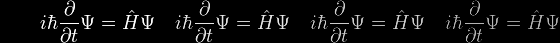# Algebra

For many people, especially as their schooldays recede further into the past, multiplication or division (or Uglification and Derision, as it has been called) of one number by another is tricky enough without a calculator.

But multiplication or division of one algebraic expression by another might be regarded as impossible or even meaningless. In fact they're not really very different at all from their numerical counterparts.

To prove that (a2 – b2) = (a – b)*(a + b) we evaluate the right-hand side by multiplying each element in the second bracket by each element in the first bracket, and then adding-up the results, remembering that 'a times b' is the same as 'b times a'.

• (a – b)*(a + b) = a*a + a*b – b*a – b*b = a*a - b*b = a2 – b2

Well that's all the homework required.

But one might well wonder if something similar holds for (a3 – b3) or (a4 – b4), and so on. And indeed it does. But we've not been given the answer in advance – in other words, we have to discover something rather than simply prove it.

In fact, we can work out (a4 – b4) straight away from what we've already proved.

• (a4 – b4) = ( (a2)2 – (b2)2 ) = (a2 – b2) * (a2 + b2) = (a – b) * (a + b) * (a2 + b2)

Indeed, we could take this a step further by introducing j which is defined as the square-root of -1.

• j = √(-1) and so j2 = -1
• (a – jb)*(a + jb) = a*a + a*jb – jb*a – j2b*b = a*a - j2b*b = a2 + b2

and so

• (a4 – b4) = (a – b) * (a + b) * (a2 + b2) = (a – b) * (a + b) *( a – jb) * (a + jb)

But this is a bit of a digression.

Cutting to the chase, we haven't yet tackled the prior case of (a3 – b3). However we feel pretty confident that it will involve (a – b). As it does.

To discover the other ingredients, we must divide (a3 – b3) by (a – b) in exactly the same way as a numerical long-division, dividing the 'a' of a – b firstly into the a3 then into the a2b and finally into the ab2, at which point the process terminates. The answer accumulates over on the right-hand side:

 a – b ) a3 – b3 ( a2 + a*b + b2 a3 – a2 b a2 b – b3 a2 b – ab2 ab2 – b3 ab2 – b3

and so

• (a3 – b3) = (a – b) * ( a2 + a*b + b2)

Exactly analogous long-divisions are possible for all higher odd powers of 'a' and 'b'

• (a5 – b5) = (a – b) * ( a4 + a3b + a2b2 + ab3 + b4)
• (a7 – b7) = (a – b) * ( a6 + a5b + a4b2 + a3b3 + a2b4 + ab5 + b6)
• etc

And indeed for all higher even powers of 'a' and 'b' too

• (a6 – b6) = (a – b) * ( a5 + a4b + a3b2 + a2b3 + ab4 + b5)
• (a8 – b8) = (a – b) * ( a7 + a6b + a5b2 + a4b3 + a3b4 + a2b5 + ab6 + b7)
• etc

but we can use the same simplifications as before

• (a6 – b6) = ((a3)2 – (b3)2) = (a3 – b3) * (a3 + b3) = (a – b) * ( a2 + a*b + b2) * (a3 + b3)
• (a8 – b8) = ((a4)2 – (b4)2) = (a4 – b4) * (a4 + b4) = (a2 – b2) * (a2 + b2) * (a4 + b4) = (a – b ) * (a + b) * (a2 + b2) * (a4 + b4)

(Eboracus just loves type-setting all this sort of stuff into html!)